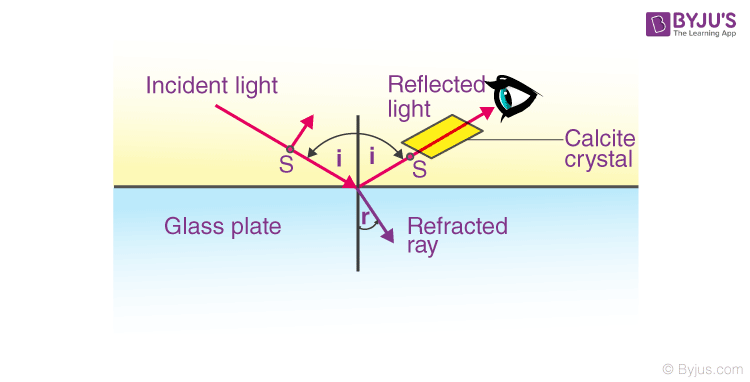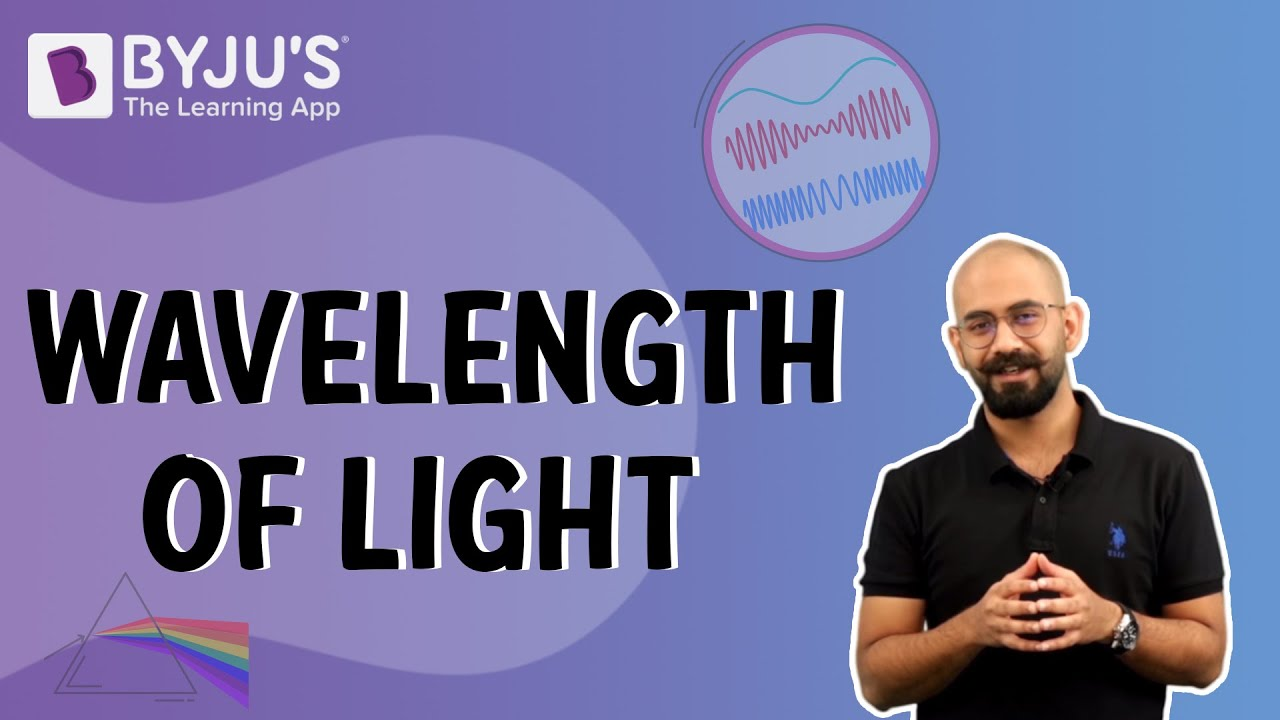# Brewster's Law

## What is Brewster’s Law?

According to Brewster’s law,

When an unpolarized light of known wavelength is incident on a transparent substance surface, it experiences maximum plan polarization at the angle of incidence whose tangent is the refractive index of the substance for the wavelength.

Brewster’s law is a relationship of light waves at the maximum polarization angle of light. This law is named after Sir David Brewster, a Scottish physicist, who proposed the law in the year 1811. The law states that the p-polarized rays vanish completely on different glasses at a particular angle.

Further, the polarization angle is also called Brewster’s angle. It is an angle of incidence where the ray of light having a p-polarization is transmitted through a dielectric surface that is transparent without any reflection. While the unpolarized light at this angle is transmitted, the light is reflected from the surface.Brewster was able to determine that the refractive index of the medium is numerically equal to the tangent angle of polarization. To know more about Brewster’s Law Formula.

$$\begin{array}{l}\mu = \tan i\end{array}$$

Where,

µ = Refractive index of the medium.

i = Polarization angle.

From Snell’s Law:

$$\begin{array}{l}\mu = \frac{\sin i}{\sin r}…….. 1\end{array}$$

From Brewster’s Law:

$$\begin{array}{l}\mu = \tan i = \frac{\sin i}{\cos i}…….. 2\end{array}$$

Comparing both formulas: 1 and 2

$$\begin{array}{l}\cos i = \sin r = \cos \left ( \frac{\pi}{2}-r \right )\end{array}$$

$$\begin{array}{l}i = \frac{\pi}{2} – r, or \ i + r = \frac{\pi}{2}\end{array}$$

$$\begin{array}{l}\textup{As,}\,\, i + r = \frac{\pi}{2} < ABC \,\, \textup{is also equal to the}\,\, \frac{\pi}{2}.\end{array}$$
Therefore, the reflected and the refracted rays are at right angles to each other.

### Relation Between Brewster Angle and Critical Angle

Brewster’s angle is given as:

Ө b = arc tan (n2/n1)

tanӨ b = n2/n1

The critical angle is given as:

Ө c = arc sin (n1/n2)

sinӨ c = n1/n2

sinӨ c = 1/(n2/n1)

But we know that,

tanӨ p = n/sub>

sinӨ c = 1/tanӨ b

Therefore,

Өc = arc sin (1/tanӨ b)

## Application of Brewster’s Law

One general example of the application of Brewster’s law is polarized sunglasses. These glasses use the principle of Brewster’s angle. The polarized glasses reduce glare that is reflected directly from the sun and also from horizontal surfaces like roads and water. Photographers also use the same law to reduce the reflection from reflective surfaces by using a polarizing filter for the lens.

### Brewster’s Law Solved Examples

Example 1:

What will be Brewster’s angle of light, if the light travels from water into the air? Assume that n = 1.33.

Solution:

Given,

n1 = 1.33

Brewster’s angle = tan-1 (n2/n1) = tan-1 (1.5/1.33) = 48.4o

Therefore, Brewster’s angle is 48.4o.

Example 2:

Determine the angle of refraction and polarization angle of the polarizer if the refractive index of the polarizer is 1.33.

Solution:

Given,

Refractive index of the polarizer = 1.33

According to Brewster’s law, μ = tan ip

ip = tan-1 (1.33)

ip = 53.06

Therefore,

Angle of refraction = ip + ir = 90 = 90 – 53.06 = 36.94